## How to Count Spaces before the Text String

This post will guide you how to count the number of spaces before the text string in a cell in Excel. How to count the leading spaces before the text string in one cell with formula in excel. How do I create an excel formula that counts the number of spaces before the text string.

## 1. Count number of spaces before a string using a Formula

Assuming that you have lots of cells contain text string with different levels of indentation on them. You want to count the number of spaces before each test string in cells, and then you want to know how to count the number of spaces before the text string in each cell.

You can create an excel formula based on the FIND function, the LEFT function, and the TRIM function.

For example, let’s count the number of spaces before text string in Cell B1, you can write down the following excel formula:

``=FIND(LEFT(TRIM(B1),1),B1)-1``

Let’s see how this formula works:

The TRIM function can be used to remove all spaces at the start or end of the text string, then you can get a text string without any spaces. The returned result will pass into the LEFT function to get the leftmost characters of the result without spaces.

Then use the FIND function to search for that characters in the original string, and it returns the position of the first character of the searching characters in the original string. The result is subtracted 1 to get the number of spaces before the text string in Cell B1.

When you use this formula, you do not need to care how many other spaces there are or where they are.

If there are no spaces at the end of the text string in each cell, then you also can use the following formula:

``=LEN(B1)- LEN(TRIM(B1))``

## 2. Count Spaces before the Text String using User Defined Function with VBA Code

You can create a user-defined function in Excel using VBA (Visual Basic for Applications) to count the number of spaces before a text string. Just do the following steps:

Step1: Press Alt + F11 to open the Visual Basic Editor.

Step2: Click Insert > Module to insert a new module.

Step3: Type the following code into the module:

```Function CountSpaces_Excelhow(str As String) As Integer
Dim i As Integer
For i = 1 To Len(str)
If Mid(str, i, 1) <> " " Then
Exit For
End If
Next i
CountSpaces_Excelhow = i - 1
End Function
```

Step4: Enter the formula into a cell, then press Enter key to apply it.

``=CountSpaces_Excelhow(B1)``

## 3. Video: Count Spaces before the Text String

This video will show you how to count spaces before the text string in Excel using a formula or VBA code.

## 4. Related Formulas

• Get first word from text string
If you want to extract the first word from a text string in a cell, you can use a combination of the IF function, the ISERR function, the LEFT function and the FIND function to create a complex excel formula..…
• Get last word from text string
If you want to get the last word from a text string, you can create an excel formula based on the RIGHT function, the LEN function, the FIND function and the SUBSTITUTE function..…
• Extract nth word from text string
If you want to extract the nth word from a text string in a single cell, you can create an excel formula based on the TRIM function, the MID function, the SUBSTITUTE function, the REPT function and the LEN function..…
• count specific words in a cell or a range
If you want to count the number of a specific word in a single cell, you need to use the SUBSTITUTE function to remove all that certain word in text string, then using LEN function to calculate the length of the substring that without that specific word.…

## 5. Related Functions

• Excel LEFT function
The Excel LEFT function returns a substring (a specified number of the characters) from a text string, starting from the leftmost character.The LEFT function is a build-in function in Microsoft Excel and it is categorized as a Text Function.The syntax of the LEFT function is as below:= LEFT(text,[num_chars])…t)…
• Excel FIND function
The Excel FIND function returns the position of the first text string (sub string) within another text string.The syntax of the FIND function is as below:= FIND(find_text, within_text,[start_num])…
• Excel LEN function
The Excel LEN function returns the length of a text string (the number of characters in a text string).The syntax of the LEN function is as below:= LEN(text)…
• Excel TRIM function
The Excel TRIM function removes all spaces from text string except for single spaces between words.  You can use the TRIM function to remove extra spaces between words in a string.The syntax of the TRIM function is as below:= TRIM (text)….

## How to Remove the First/Last Word from Text string in Cell

This post will guide you how to remove the first and the last word from a text string in cells using a formula or User defined function with VBA code in Excel 2013/2016/2019/365. How do I use a formula to remove first and last word of a text string in Excel.

## 1. Remove the First Word from Text String using Formula

If you want to remove the first word from a text string in cells in Excel, you can use a formula based on the RIGHT function, the LEN function and the FIND function. Like this:

``=RIGHT(B1,LEN(B1)-FIND(" ",B1))``

Type this formula into a blank cell and press Enter key on your keyboard and then drag the AutoFill Handle down to other cells to apply this formula.

## 2. Remove the Last Word from Text String using Formula

If you want to remove the last word from a text string, you can use a formula based on the LEFT function, the TRIM function, the FIND function, and the SUBSTITUTE function. Like this:

``=LEFT(TRIM(B1),FIND("~",SUBSTITUTE(B1," ","~",LEN(TRIM(B1))-LEN(SUBSTITUTE(TRIM(B1)," ",""))))-1)``

Type this formula into a blank cell and press Enter key on your keyboard and then drag the AutoFill Handle down to other cells to apply this formula.

## 3. Remove the First Word from Text String using User Defined Function with VBA Code

You can create a User Defined Function in VBA to remove the first word from a text string in Excel by following these steps:

Step1: Open the Visual Basic Editor (VBE) by pressing Alt+F11.

Step2: In the VBE, click on Insert -> Module to create a new module.

Step3: In the module, enter the following code:

```Function RemoveFirstWord_ExcelHow(ByVal inputString As String) As String
Dim firstSpaceIndex As Integer
firstSpaceIndex = InStr(1, inputString, " ")
If firstSpaceIndex > 0 Then
RemoveFirstWord_ExcelHow = Mid(inputString, firstSpaceIndex + 1, Len(inputString))
Else
RemoveFirstWord_ExcelHow = ""
End If

End Function```

Step5: In a blank cell, enter the following formula:

``=RemoveFirstWord(B1)``

Where B1 is the cell that contains the text string you want to remove the first word from.

Step6: Press Enter to display the result.

## 4. Remove the Last Word from Text String using User Defined Function with VBA Code

If you also want to remove the last word from a text string in excel using a User Defined Function with VBA code, and you can refer to the above steps, and just using the following code:

```Function RemoveLastWord_ExcelHow(ByVal inputString As String) As String
Dim lastSpaceIndex As Integer
lastSpaceIndex = InStrRev(inputString, " ")
If lastSpaceIndex > 0 Then
RemoveLastWord_ExcelHow = Left(inputString, lastSpaceIndex - 1)
Else
RemoveLastWord_ExcelHow = ""
End If
End Function```

In Cell E1, type the following formula, press Enter key to apply it:

``=RemoveLastWord_ExcelHow(B1)``

## 5. Video: Remove the First/Last Word from Text string in Cell

This video will demonstrate how to remove the first/last word from a text string using a formula and VBA code.

## 6. Related Functions

• Excel LEFT function
The Excel LEFT function returns a substring (a specified number of the characters) from a text string, starting from the leftmost character.The LEFT function is a build-in function in Microsoft Excel and it is categorized as a Text Function.The syntax of the LEFT function is as below:= LEFT(text,[num_chars])…t)…
• Excel FIND function
The Excel FIND function returns the position of the first text string (sub string) within another text string.The syntax of the FIND function is as below:= FIND(find_text, within_text,[start_num])…
• Excel RIGHT function
The Excel RIGHT function returns a substring (a specified number of the characters) from a text string, starting from the rightmost character.The syntax of the RIGHT function is as below:= RIGHT (text,[num_chars])…
• Excel Substitute function
The Excel SUBSTITUTE function replaces a new text string for an old text string in a text string.The syntax of the SUBSTITUTE function is as below:= SUBSTITUTE  (text, old_text, new_text,[instance_num])….
• Excel LEN function
The Excel LEN function returns the length of a text string (the number of characters in a text string).The syntax of the LEN function is as below:= LEN(text)…
• Excel TRIM function
The Excel TRIM function removes all spaces from text string except for single spaces between words.  You can use the TRIM function to remove extra spaces between words in a string.The syntax of the TRIM function is as below:= TRIM (text)….

## Extract Email Address from Text

This post will guide you how to extract email address from a text string in Excel. How do I use a formula to extract email address in Excel. How to extract email address from text string with VBA Macro in Excel.

Assuming that you have a list of data in range B1:B5 which contain text string and you want to extract all email addresses from those text string. How to achieve it. You can use a formula or VBA Macro to achieve the result. Let’s see the below introduction.

## 1. Extract Email Address from Text with a Formula

To extract email address from text string in cells, you can use a formula based on the TRIM function, the RIGHT function, the SUBSTITUTE function, the LEFT function, the FIND function, the REPT function and the LEN function. Just like this:

``=TRIM(RIGHT(SUBSTITUTE(LEFT(B1,FIND(" ",B1&" ",FIND("@",B1))-1)," ",REPT(" ",LEN(B1))),LEN(B1)))``

Select the adjacent Cell C1, and type this formula, and press Enter key in your keyboard, and then drag the AutoFill Handle over other cells to apply this formula.

## 2. Extract Email Address from Text with User Defined Function

You can also write a User Defined Function with VBA Code to extract email address quickly, just do the following steps:

Step1: open your excel workbook and then click on “Visual Basic” command under DEVELOPER Tab, or just press “ALT+F11” shortcut.

Step2: then the “Visual Basic Editor” window will appear.

Step3: click “Insert” ->”Module” to create a new module.

Step4: paste the below VBA code into the code window. Then clicking “Save” button.

```Function ExtractEmailFromText(s As String) As String
Dim AtTheRateSignSymbol As Long
Dim i As Long
Dim TempStr As String
Const CharList As String = "[A-Za-z0-9._-]"

AtTheRateSignSymbol = InStr(s, "@")
If AtTheRateSignSymbol = 0 Then
ExtractEmailFromText = ""
Else
TempStr = ""
For i = AtTheRateSignSymbol - 1 To 1 Step -1
If Mid(s, i, 1) Like CharList Then
TempStr = Mid(s, i, 1) & TempStr
Else
Exit For
End If
Next i

If TempStr = "" Then Exit Function

TempStr = TempStr & "@"

For i = AtTheRateSignSymbol + 1 To Len(s)
If Mid(s, i, 1) Like CharList Then
TempStr = TempStr & Mid(s, i, 1)
Else
Exit For
End If
Next i
End If

If Right(TempStr, 1) = "." Then TempStr = Left(TempStr, Len(TempStr) - 1)

ExtractEmailFromText = TempStr
End Function

```

Step5: Type the following formula into blank cells and then press Enter key.

``=ExtractEmailFromText(B1)``

Type this formula into a blank cell and then press Enter key in your keyboard.

Step6: lets see the result:

## 3. Video: Extract Email Address from Text in Excel

This video will demonstrate a step-by-step instruction on how to use the formula and VBA code to extract email addresses from a block of text in Excel, making it easy to manage and organize your contact information.

## How to extract text after the second or nth specific character (space or comma)

In the previous post, we talked that how to extract text after the first occurrence of the comma character in excel. And this post explains that how to get a substring after the second or nth occurrence of the comma or space character in excel.

## 1. Extract Text after The Second or Nth Specific Character

If you want to extract text after the second or nth comma character in a text string in Cell B1, you need firstly to get the position of the second or nth occurrence of the comma character in text.

So you can use the SUBSTITUTE function to replace the second comma character with the hash character, then using the FIND function to look for the first hash character in text returned by substitute function, the returned value is the position of the second occurrence of the comma character in text.

Last, you can use the MID function to extract a substring that you want to extract.

So you can create a formula based on the MID function, the FIND function and the SUBSTITUTE function as follows:

``=TRIM(MID(B1,FIND("#",SUBSTITUTE(B1,",","#",2))+1,255))``

Let’s see how this formula works:

=SUBSTITUTE(B1,”,”,”#”,2)

This function replaces the second comma character with hash character in cell B1, and the returned result goes into the FIND function as its within_text argument.

=FIND(“#”,SUBSTITUTE(B1,”,”,”#”,2))+1

The FIND function will return the position of the first occurrence of the hash character in within_text string returned by the SUBSTITUTE function. Then add 1 to get the starting position after the second occurrence of the comma character in text. And it returns 11. It is fed into the MID function as its start_num argument.

=MID(B1,FIND(“#”,SUBSTITUTE(B1,”,”,”#”,2))+1,255)

The MID function will extracts 255 characters from a text string in Cell B1, starting at the 11character.

=TRIM()

The TRIM function removes all extra space characters and just leave only one between words.

If you want to extract a substring after the third occurrence of the comma character, you can use the following formula:

``=TRIM(MID(B1,FIND("#",SUBSTITUTE(B1,",","#",3))+1,255))``

## 2. Extract Text after The Second or Nth Specific Character using User Defined Function with VBA

You can use the VBA Split function to split a string into an array of substrings based on a specified delimiter, and then extract the desired substring based on its index in the array.

You can use a user-defined function that extracts the substring after the third occurrence of the comma character, just do the following steps:

Step1: Press the Alt + F11 keys on your keyboard to open the VBA Editor.

Step2: In the VBA Editor, insert a new module by clicking on “Insert” in the top menu and then selecting “Module“.

Step3: Copy the VBA code that you want to run and paste it into the new module.

Step4: Save the module by clicking on “File” in the top menu and then selecting “Save“.

Step5: in a blank cell, type the following formula:

``=ExtractSubstring_ExcelHow(B1)``

Step6: Press Enter to apply the formula and see the result in the given cell.

The function should now be applied to the selected cell and the output should be the desired substring after the third occurrence of the comma character.

## 3. Video: Extract Text after The Second or Nth Specific Character

This video will show you how to extract a substring after the third occurrence of the comma character in Excel using both a formula and VBA code.

## 4. Related Formulas

• Extract Text between Parentheses
If you want to extract text between parentheses in a cell, then you can use the search function within the MID function to create a new excel formula…
• Extract Text between Brackets
If you want to extract text between brackets in a cell, you need to create a formula based on the SEARCH function and the MID function….
• Extract Text between Commas
To extract text between commas in Cell B1, you can use the following formula based on the SUBSTITUTE function, the MID function and the REPT function…..
• Extract word that starting with a specific character
Assuming that you have a text string that contains email address in Cell B1, and if you want to extract word that begins with a specific character “@” sign, you can use a combination with the TRIM function, the LEFT function, the SUBSTITUTE function ….
• Extract text before first comma or space
If you want to extract text before the first comma or space character in cell B1, you can use a combination of the LEFT function and FIND function….
• Extract text after first comma or space
If you want to get substring after the first comma character from a text string in Cell B1, then you can create a formula based on the MID function and FIND function or SEARCH function ….
• Extract text before the second or nth specific character
you can create a formula based on the LEFT function, the FIND function and the SUBSTITUTE function to Extract text before the second or nth specific character…

## 5. Related Functions

• Excel MID function
The Excel MID function returns a substring from a text string at the position that you specify.The syntax of the MID function is as below:= MID (text, start_num, num_chars)….
• Excel FIND function
The Excel FIND function returns the position of the first text string (sub string) within another text string.The syntax of the FIND function is as below:= FIND(find_text, within_text,[start_num])…
• Excel SEARCH function
The Excel SEARCH function returns the number of the starting location of a substring in a text string.The syntax of the SEARCH function is as below:= SEARCH  (find_text, within_text,[start_num])…
• Excel Substitute function
The Excel SUBSTITUTE function replaces a new text string for an old text string in a text string.The syntax of the SUBSTITUTE function is as below:= SUBSTITUTE  (text, old_text, new_text,[instance_num])….
• Excel TRIM function
The Excel TRIM function removes all spaces from text string except for single spaces between words.  You can use the TRIM function to remove extra spaces between words in a string.The syntax of the TRIM function is as below:= TRIM (text)….

## How to Reverse Concatenate Formula in Excel

If you have ever needed to split text that was combined using the concatenate function, and you may be want to know if there is a way to reverse the process and spearate the text into its orginal form.

This post will guide you how to reverse the concatenate function using a formula in Excel, as well as “Text to Columns” feature. How do I split the text string into the muliptle cells with a formula in Excel. How to split text data of a cell to multiple cells in Excel.

## 1. Video: How to Reverse Concatenate Formula in Excel

This video tutorial demonstrates the steps for using a formula and the “Text to Columns” feature to split text in Excel.

## 2. Reverse Concatenate Formula

To reverse concatenate formula in Excel, you can use a formula based on the TRIM function, the MID function, the SUBSTITUTE function, the REPT function, and the COLUMNS function.
Assuming that you have a list of data in range B1:B4 that contain text string, and you want to split the string by comma character. you can write down the following formula:

``=TRIM(MID(SUBSTITUTE(\$B1,",",REPT(" ",999)),COLUMNS(\$A:A)*999-998,999))``

Type this formula into a blank cell and then press Enter key and then drag the Fill Handle down to other cells to apply this formula.

You will see that all text string in range of cells B1:B4 have been split into multiple cells by a comma character.

This method can be useful for working with data that has been combined using the concatenate function or for importing data from other sources that may be in a combined format.

## 3. Split text string to Multiple Cells with Text To Column Feature

The opposite of concatenate in excel is to split or separate text that have been combined using the concatenate function. If you want to split text into separate cells in excel, you can also use the Text To column Feature to reverse the concatenate formula to split text string in the range of cells B1:B4 to multiple cells in Excel. Just do the following steps:
Step1: select the range of cells that you want to reverse.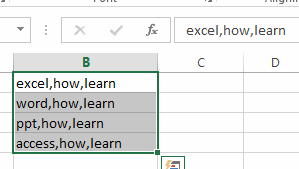Step2: go to DATA tab, click Text To column command under Data Tools group. and the Convert  Text to Columns Wizard dialog will open.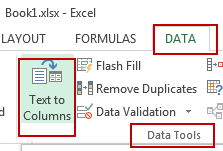Step3: select Delimited option in the Original data type section, then click Next  button.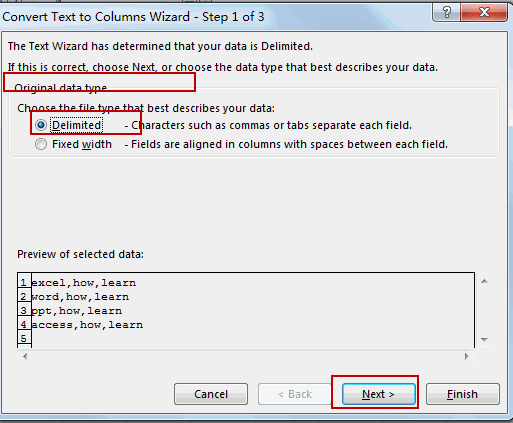Step4: check Other checkbox, and type the delimiter that you want to use, such as: a comma character. and then click Next button.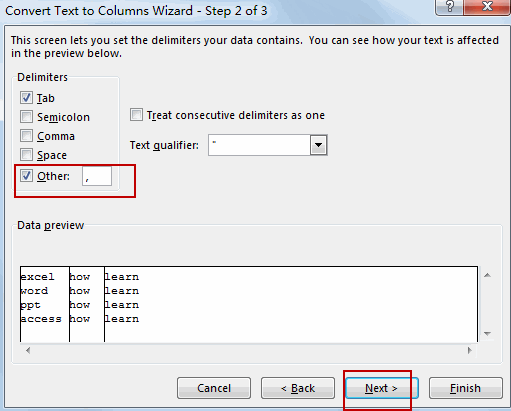Step5: select General option under column data format section, and select a destination cell where you want to place the split results.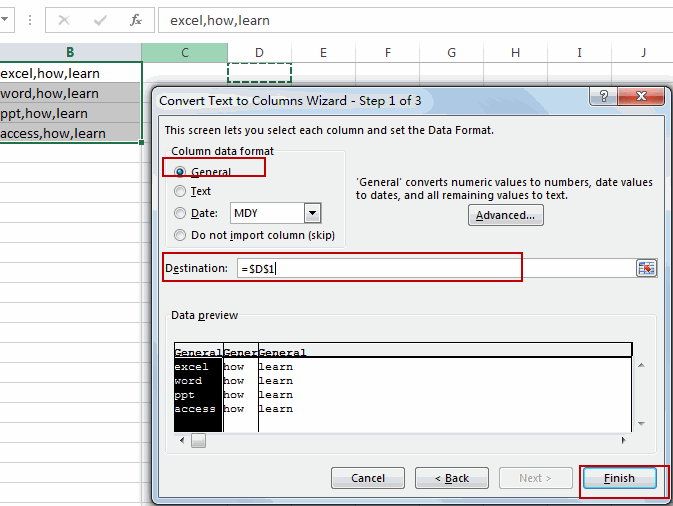Step6: Once you are satisfied with the preview, click on “Finish” to split the text into separate columns.

Your text will now be split into separate columns based on the delimiter or width you specified.

## 4. Related Functions

• Excel Substitute function
The Excel SUBSTITUTE function replaces a new text string for an old text string in a text string.The syntax of the SUBSTITUTE function is as below:= SUBSTITUTE  (text, old_text, new_text,[instance_num])….
• Excel TRIM function
The Excel TRIM function removes all spaces from text string except for single spaces between words.  You can use the TRIM function to remove extra spaces between words in a string.The syntax of the TRIM function is as below:= TRIM (text)….
• Excel MID function
The Excel MID function returns a substring from a text string at the position that you specify.The syntax of the MID function is as below:= MID (text, start_num, num_chars)….
• Excel REPT function
The Excel REPT function repeats a text string a specified number of times.The syntax of the REPT function is as below:= REPT  (text, number_times)…
• Excel COLUMNS function
The Excel COLUMNS function returns the number of columns in an Array or a reference.The syntax of the COLUMNS function is as below:=COLUMNS (array)….

## Abbreviate Names Or Words in Excel

As an MS Excel user, you might have come across a task where you need to abbreviate different names or words, and there are also possibilities that you might have done this task manually by assuming that there isn’t any other way to do this task, but you assumed wrong because fortunately there is a way which would let you abbreviate names and words in a matter of seconds unlike doing it manually which consumes a lot of time and leaves you with no satisfying results.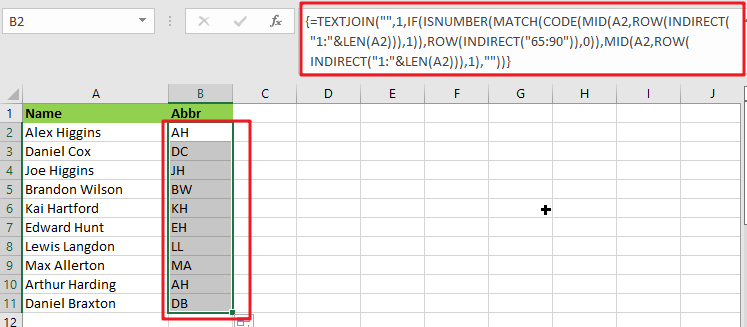So for exploring that way/method, let’s dive into the article.

## General Formula:

The array formula to abbreviate different names and words in few seconds is mentionedas follows:

`{=TEXTJOIN("",1,IF(ISNUMBER(MATCH(CODE(MID(A2,ROW(INDIRECT("1:"&LEN(A2))),1)),ROW(INDIRECT("65:90")),0)),MID(A2,ROW(INDIRECT("1:"&LEN(A2))),1),""))}`## Syntax Explanation:

To understand the working of the above formula, we first need to know about each syntax and how they contribute to abbreviating names and words in seconds.

• `TEXTJOIN`: The TEXTJOIN function in Microsoft Excel allows you to join two or more strings together, with each value separated by a delimiter. The TEXTJOIN function is an Excel built-in function classified as a String/Text Function.
• `IF`: The IF function is one of Excel’s most used functions, allowing you to create logical comparisons between a number and what you anticipate.
• `ISNUMBER`: When a cell contains a number, the ISNUMBER function returns TRUE; otherwise, it returns FALSE. ISNUMBER can be used to verify that a cell contains a numeric value or that the output of another function is a number.
• `MATCH`: The MATCH is a function used to find a lookup value in a row, column, or table in MS Excel. MATCH allows for approximate and accurate matching and wildcards (*?) for partial matches.
• `CODE`: The CODE function in Excel returns a numeric code for a specified character.
• INDIRECT: The INDIRECT function returns a range reference. This function may generate a reference that will not change if a row or column is added to the worksheet.

## Let’s See How This Formula Works:

To abbreviate capital letters text, use this array formula based on the TEXTJOIN function, new in Office 365 and Excel 2019. You may use this method to generate initials from names or acronyms. Because only capital letters can survive this algorithm, the original text must contain capitalized terms. If necessary, you can capitalize words using the PROPER function.

The formula in B2 in the case provided is:

`=TEXTJOIN("",1,IF(ISNUMBER(MATCH(CODE(MID(A2,ROW(INDIRECT("1:"&LEN(A2))),1)),ROW(INDIRECT("65:90")),0)),MID(A2,ROW(INDIRECT("1:"&LEN(A2))),1),""))`

The MID function is used to turn the string into an array of individual letters from the inside out:

`=MID(A2,ROW(INDIRECT("1:"&LEN(A2))),1)`

In this formula section, the operators MID, ROW, INDIRECT, and LEN transform a string into an array of letters.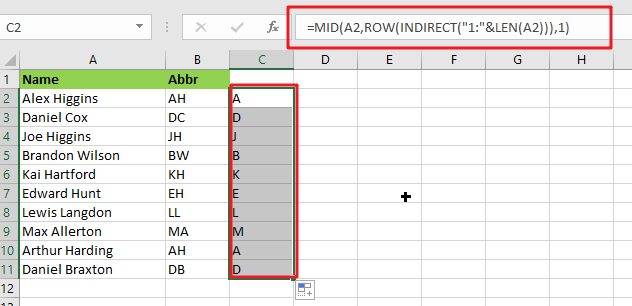MID delivers an array of all characters in the text.

`=CODE(MID(A2,ROW(INDIRECT("1:"&LEN(A2))),1))`

This above array is sent to the CODE function, which returns an array of numeric ASCII codes, one for each letter.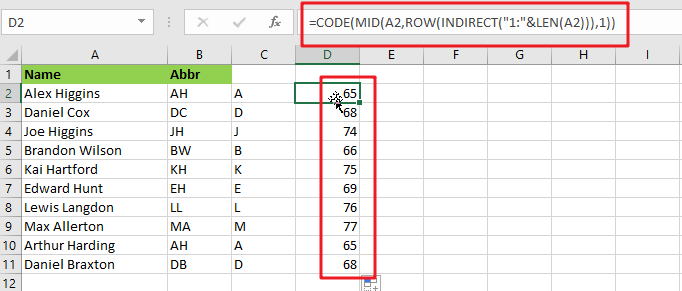`=ROW(INDIRECT("65:90")`

ROW and INDIRECT are used to generate another numeric array:

`{65;66;67;68;69;70;71;72;73;74;75;76;77;78;79;80;81;82;83;84;85;86;87;88;89;90}`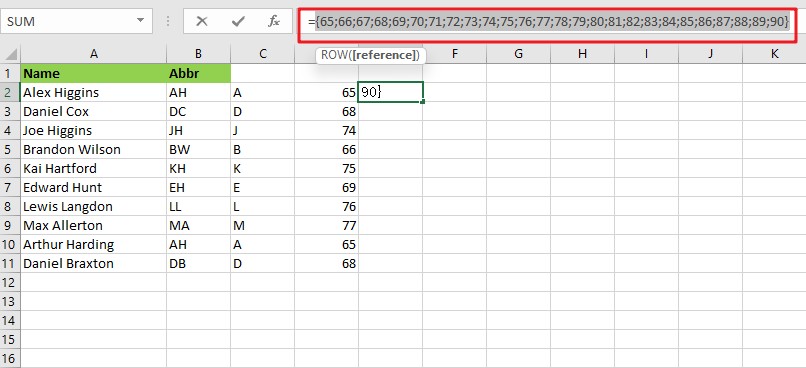Note: The digits 65 to 90 correspond to the ASCII codes for all capital letters from A to Z. This array is used as the lookup array in the MATCH function, and the original array of ASCII codes is given as the lookup value.

`{=MATCH(CODE(MID(A2,ROW(INDIRECT("1:"&LEN(A2))),1)),ROW(INDIRECT("65:90")),0)}`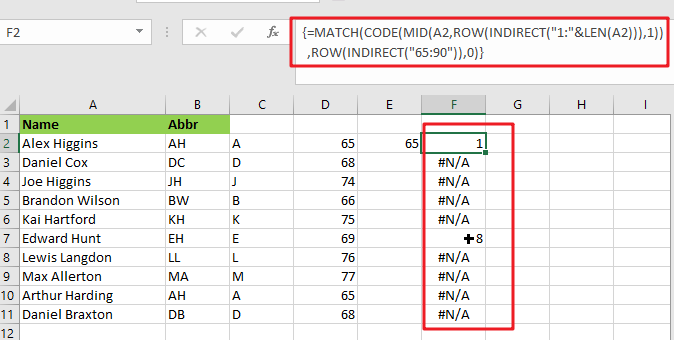The MATCH function returns either a number or the #N/A error. Because numbers represent capital letters.

`{=IF(ISNUMBER(MATCH(CODE(MID(A2,ROW(INDIRECT("1:"&LEN(A2))),1)),ROW(INDIRECT("65:90")),0)),MID(A2,ROW(INDIRECT("1:"&LEN(A2))),1),"")}`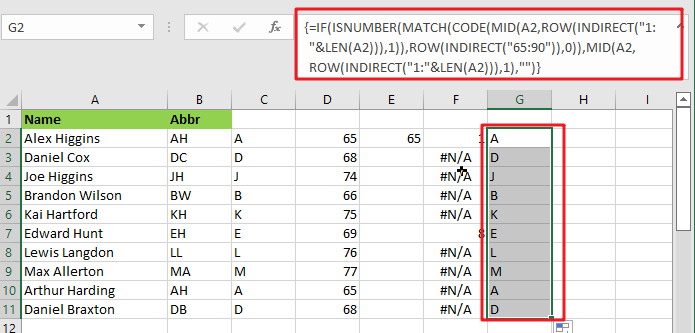The ISNUMBER function is used with the IF function to filter results. Only characters with ASCII codes between 65 and 90 will be included in the final array, reconstructed using the TEXTJOIN method to produce the final abbreviation or acronym.

## Abbreviate Names And Words In MS Excel 2016 Or Older Versions

As in Excel 2016 and earlier versions, the formula discussed above would not work, so the TRIM function is available in Excel 2016 and earlier versions, so we use it to abbreviate names and words.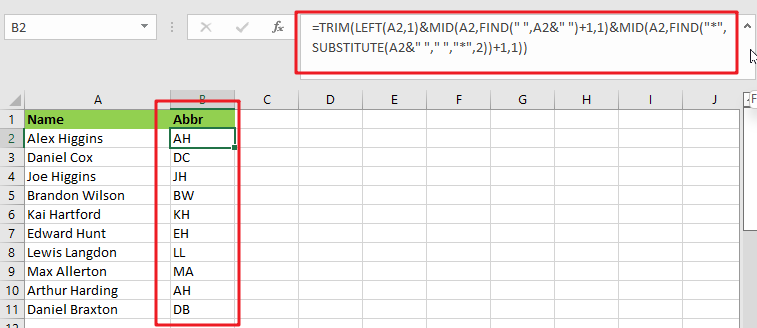### General Formula:

The formula we would use in MS Excel 2016 and earlier versions to abbreviate names and words as follows.

`=TRIM(LEFT(Text,1)&MID(Text,FIND(" ",Text&" ")+1,1)&MID(Text,FIND("*",SUBSTITUTE(Text&" ","*,"2))+1,1)`

### How Does This Formula Work?

From cell A2, if you want to extract the initials, enter this formula in cell B2.

`=TRIM(LEFT(A2,1)&MID(A2,FIND(" ",A2&" ")+1,1)&MID(A2,FIND("*",SUBSTITUTE(A2&" "," ","*",2))+1,1))`

Here the text string is the string you want to extract the first letters of each word.

When you press the Enter key, all of the first letters of each word in cell A2 are retrieved.### Explanation:

1. The TRIM function eliminates any extra spaces from the text string.
2. The LEFT(A2,1) function retrieves the first letter of the text string.
3. MID(A2,FIND(” “,A2&” “)+1,1) retrieves the initial letter of the second word separated by a space.
4. MID(A2,FIND(“*”,SUBSTITUTE(A2&” “,” “,”*,”2))+1,1) retrieves the initial letter of the third word separated by a space.

NOTE:

This formula only works when three or fewer words are in a cell. You can modify ” “ in the formula to different delimiters.

This formula extracts the initial characters in a case-insensitive manner; if you want the formula to always return in the upper case, include the UPPER function in the formula.

`=UPPER(TRIM(LEFT(A2,1)&MID(A2,FIND(" ",A2&" ")+1,1)&MID(A2,FIND("*",SUBSTITUTE(A2&" "," ","*",2))+1,1)))`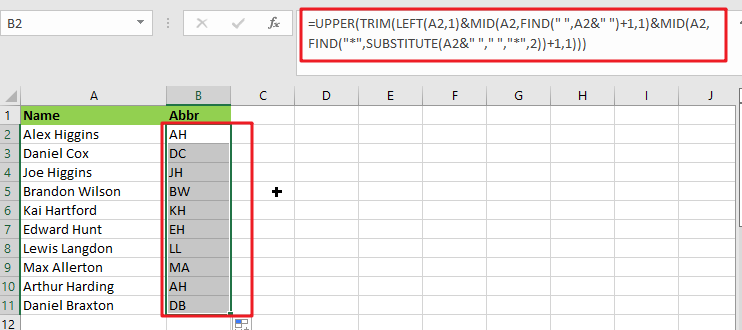### Related Functions

• Excel IF function
The Excel IF function perform a logical test to return one value if the condition is TRUE and return another value if the condition is FALSE. The IF function is a build-in function in Microsoft Excel and it is categorized as a Logical Function.The syntax of the IF function is as below:= IF (condition, [true_value], [false_value])….
• Excel TEXTJOIN function
The Excel TEXTJOIN function joins two or more text strings together and separated by a delimiter. you can select an entire range of cell references to be combined in excel 2016.The syntax of the TEXTJOIN function is as below:= TEXTJOIN  (delimiter, ignore_empty,text1,[text2])…
• Excel ROW function
The Excel ROW function returns the row number of a cell reference.The ROW function is a build-in function in Microsoft Excel and it is categorized as a Lookup and Reference Function.The syntax of the ROW function is as below:= ROW ([reference])….
• Excel MID function
The Excel MID function returns a substring from a text string at the position that you specify.The syntax of the MID function is as below:= MID (text, start_num, num_chars)…
• Excel INDIRECT function
The Excel INDIRECT function returns the cell reference based on a text string, such as: type the text string “A2” in B1 cell, it just a text string, so you can use INDIRECT function to convert text string as cell reference….
• Excel MATCH  function
The Excel MATCH function search a value in an array and returns the position of that item.The MATCH function is a build-in function in Microsoft Excel and it is categorized as a Lookup and Reference Function.The syntax of the MATCH function is as below:= MATCH  (lookup_value, lookup_array, [match_type])….
• Excel ISNUMBER function
The Excel ISNUMBER function returns TRUE if the value in a cell is a numeric value, otherwise it will return FALSE.The syntax of the ISNUMBER function is as below:= ISNUMBER (value)…
• Excel CODE function
The Excel CODE function returns the numeric ASCII value for the first character of a text string.The syntax of the CODE function is as below:= CODE  (text)…
• Excel LEN function
The Excel LEN function returns the length of a text string (the number of characters in a text string).The LEN function is a build-in function in Microsoft Excel and it is categorized as a Text Function.The syntax of the LEN function is as below:= LEN(text)…
• Excel LEFT function
The Excel LEFT function returns a substring (a specified number of the characters) from a text string, starting from the leftmost character.The LEFT function is a build-in function in Microsoft Excel and it is categorized as a Text Function.The syntax of the LEFT function is as below:= LEFT(text,[num_chars])…t)…
• Excel FIND function
The Excel FIND function returns the position of the first text string (sub string) within another text string.The syntax of the FIND function is as below:= FIND(find_text, within_text,[start_num])…
• Excel TRIM function
The Excel TRIM function removes all spaces from text string except for single spaces between words.  You can use the TRIM function to remove extra spaces between words in a string.The syntax of the TRIM function is as below:= TRIM (text)….

## Extract Multiple Lines From A Cell

Suppose that you have listed some text in a single cell which is separated by the line break(you can do it by pressing `ALT + ENTER` after entering the text), and now you want to extract multiple lines of text from a single cell into a separate cell, just like it has been done in the screenshot below:

You would now think that you can do it manually, but keep it into your consideration that it seems easy when there are one or two cells from which you want to extract multiple lines of text into the separate cells, but when it comes to multiple cells from which you need to extract multiple lines of text into the separate cells then doing it manually would be the foolish attempt because by this you would get tired of it and would never complete your work on time.

But don’t be worry about it because after carefully reading this article, extracting multiple lines of text into separate cells would become a piece of cake for you.

So let’s dive into the article to take you out of this fix.

## General Formula:

The Following formula would help you out for extracting multiple lines of text into seprate cells:

`=TRIM(MID(SUBSTITUTE(B1,CHAR(10),REPT(” “,LEN(B1))), (N-1)*LEN(B1)+1, LEN(B1)))`

or

`=TRIM(MID(SUBSTITUTE(\$B1,CHAR(10),REPT(" ",LEN(\$B1))), (C\$1-1)*LEN(\$B1)+1, LEN(\$B1)))`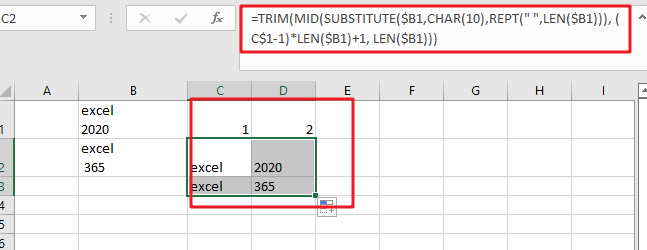## Syntax Explanations:

Before going into the explanation of the formula for getting the work done efficiently, we must understand each syntax which would make it easy for you that how each syntax contributes to extracting the multiple lines of text into the separate cells:

• `TRIM`: This function contributes to removing the extra spaces from the text, whether the space would be at the start or at the end of the text string.
• `REPT`: In MS Excel, the REPT function is used to repeat characters to a given number of times.
• `MID`: The MID function contributes to extracting the number (beginning from the left side) or characters from the given string.
• `FIND`: In MS Excel, the MID function contributes to finding out the specific text string inside the other.
• `LEN`: In MS Excel, the LEN functioncontributes to finding out the length of the text string.
• `SUBSTITUTE`: This function replaces the existing text with new text in a text string when you want to replace the text based on its content, not on its position.
• `B1`: It contributes to representing the input value.
• `Comma symbol (,)`: This comma symbol acts as a separator that separates the list of values.
• `Parenthesis ()`: The primary purpose of this parenthesis symbol is to group the various elements.
• `Minus Operator (-)`: This minus symbol contributes to subtracting any two values.
• `Plus operator (+)`: This plus symbol adds the values.

## Let’s See How This Formula Works:

The main point is that using the SUBSTITUTE and REPT functions, this formula searches for a line delimiter (“delim”) and then replaces it with many spaces.

Note that if you are using Excel on Mac and if your Excel version is old, then instead of using “CHAR(10)”, you should use “CHAR(13)” as for returning a character based upon its numeric code CHAR function is used.

The overall length of the text in the cell determines the number of spaces needed to replace the line delimiter. The formula then uses the MID function to extract the desired line. The following snippet of the formula carries out the starting point

(N-1)*LEN(B1)+1

In the above snippet, the “N” stands for “nth line,” which is picked up with the reference from the 1th row. The snippet “ LEN(B1) “ is used to identify the total number of characters extracted and is definitely equal to the length of the overall text string.

Now, to trim out all the extra space characters and to return just the line text, the TRIM function is used.

## Built-In Text to Columns Feature:

Moreover, you should know about it that there is also a built-in feature in MS Excel named Text to Columns feature (achieved by pressing Control + J ) that splits up the text according to the delimiter of your choice as if you are thinking to use this built-in way for extract multiple lines of text from a single cell into the separate cell instead of the formula explained above then stop here, as this built-in feature is not appreciated over the formula by the professionals because with the use of this built-in feature results are not more concise or desirable than the results after using the formula.

### Related Functions

• Excel TRIM function
The Excel TRIM function removes all spaces from text string except for single spaces between words. The syntax of the TRIM function is as below:=TRIM(text)…
• Excel MID function
The Excel MID function returns a substring from a text string at the position that you specify.The syntax of the MID function is as below:= MID (text, start_num, num_chars)…
• Excel Substitute function
The Excel SUBSTITUTE function replaces a new text string for an old text string in a text string. The syntax of the SUBSTITUTE function is as below:= SUBSTITUTE  (text, old_text, new_text,[instance_num])….
• Excel CHAR function
The Excel CHAR function returns the character specified by a number (ASCII Value).The CHAR function is a build-in function in Microsoft Excel and it is categorized as a Text Function. The syntax of the CHAR function is as below:=CHAR(number)….
• Excel REPT function
The Excel REPT function repeats a text string a specified number of times.The REPT function is a build-in function in Microsoft Excel and it is categorized as a Text Function.The syntax of the REPT function is as below:= REPT  (text, number_times)….
• Excel LEN function
The Excel LEN function returns the length of a text string (the number of characters in a text string).The LEN function is a build-in function in Microsoft Excel and it is categorized as a Text Function.The syntax of the LEN function is as below:= LEN(text)…

## How to Remove All Extra Spaces and Keep Only One Between Words in Excel

Sometimes when copying something from other type files to excel, there might be two or more spaces display between words. Extra spaces between words are frequently to be seen in excel, and this behavior is very annoying. If we want to make words looks neatly and make only one space between them, we need to find a simple way to remove these spaces totally, otherwise remove them by manual is very troublesome. So, in this article, we will provide you two ways to remove extra spaces conveniently. The first way, you can apply TRIM function to remove extra spaces; the second way, you can via editing VBA macro to implement removing spaces.

Precondition:

See screenshot below. There are extra spaces between words. If we want to only keep one space between words like normal, we need to remove the extra ones.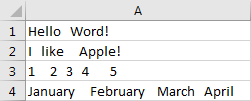## Method 1: Remove Extra Spaces by Formula (TRIM Function)

We will introduce you a simple function in excel, it is TRIM. TRIM function can remove all spaces and leave only one single space between words, that means if there is only one space between text, this space will not be removed. Now let’s see how to use TRIM to remove spaces in below steps.

Step 1: Select a blank cell for example B1, enter the formula =TRIM(A1).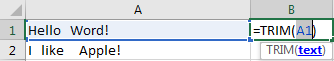Step 2: Press Enter. Verify that ‘Hello Word!’ is displayed.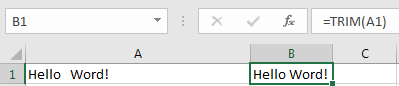Step 3: Drag down the fill handle to the end. Verify that extra spaces are removed properly.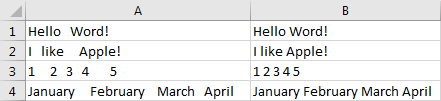## Method 2: Remove Extra Spaces by VBA Macro

Step 1: Enter Microsoft Visual Basic for Applications window via Developer->Visual Basic. You can also press Alt + F11 keys simultaneously to open it.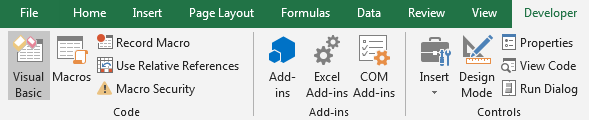Step 2: In Microsoft Visual Basic for Applications window, click Insert->Module, enter below code in Module1:

```Sub RemoveExtraSpaces()

Dim ActiveRange As Range

Dim SelectRange As Range

On Error Resume Next

xTitleId = "Remove Extra Space"

Set SelectRange = Application.Selection

Set SelectRange = Application.InputBox("Select Range", xTitleId, SelectRange.Address, Type:=8)

For Each ActiveRange In SelectRange

ActiveRange.Value = Application.WorksheetFunction.Trim(ActiveRange.Value)

Next

End Sub```

Step 3: Save VBA macro, see screenshot below. And then quit Microsoft Visual Basic for Applications.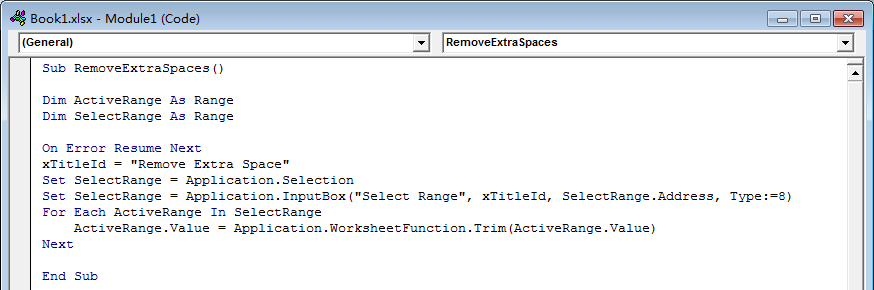Step 4: Click Developer->Macros to run Macro. Select ‘RemoveExtraSpaces’ and click Run.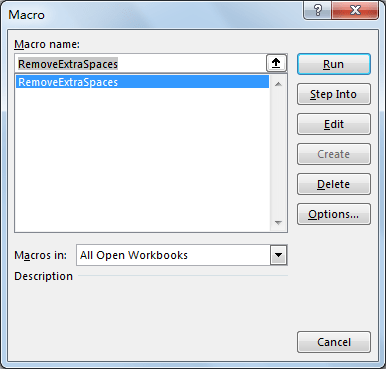Step 5: Remove Extra Space dialog pops up. Enter Select Range \$A\$1:\$A\$4. Click OK. In this step you can select the range you want to remove extra spaces.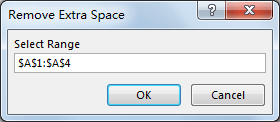Step 6: After clicking OK, check the result. Verify that extra spaces are removed, only one single space keeps between text.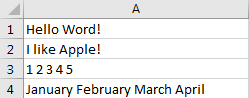Related Functions

• Excel TRIM function
The Excel TRIM function removes all spaces from text string except for single spaces between words.  You can use the TRIM function to remove extra spaces between words in a string.The syntax of the TRIM function is as below:= TRIM (text)….

## How to Remove Middle Name from Full Name in Excel

When we get a list of full names, we can remove the middle name for short in some cases. As the middle names are different among the full name list so we cannot replace them by space directly in excel. This article will provide you two methods to remove the middle name. User can use Formula to remove the middle name, or can remove it by Find and Replace function.

Prepare a list of full names.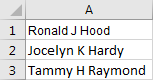## Remove the Middle Name from Full Name by Formula

Step1: Select a blank cell next to the full name, for example select B1. Enter the following formula into B1:

=TRIM(LEFT(A1,FIND(” “,LOWER(A1),1))) & ” ” & TRIM(MID(A1,FIND(” “,LOWER(A1),FIND(” “,LOWER(A1),1)+1)+1,LEN(A1)-FIND(” “,LOWER(A1),1)+1)) .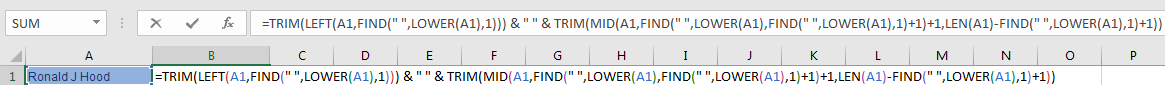Step2: Click Enter and get the result.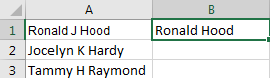Step3: Drag the Auto Fill handle to fill B2 and B3. Now all the middle names are removed from the list.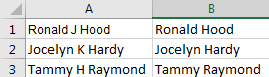## Remove the Middle Name from Full Name by Find and Replace Function

Step1: Click Ctrl+F to load Find and Replace window.

Step2: Under Replace tab, in Find what textbox enter ‘ * ‘ (a space + * + a space); in Replace with textbox enter ‘ ‘ (a space). Then click Replace All.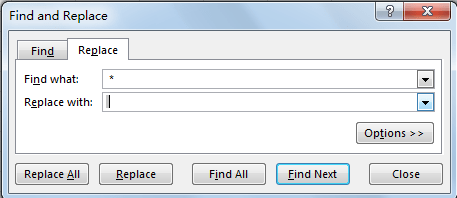Step3: Check the result. All the middle names are removed.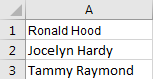### Related Functions

• Excel LEFT function
The Excel LEFT function returns a substring (a specified number of the characters) from a text string, starting from the leftmost character.The LEFT function is a build-in function in Microsoft Excel and it is categorized as a Text Function.The syntax of the LEFT function is as below:= LEFT(text,[num_chars])…t)…
• Excel FIND function
The Excel FIND function returns the position of the first text string (sub string) within another text string.The syntax of the FIND function is as below:= FIND(find_text, within_text,[start_num])…
• Excel TRIM function
The Excel TRIM function removes all spaces from text string except for single spaces between words.  You can use the TRIM function to remove extra spaces between words in a string.The syntax of the TRIM function is as below:= TRIM (text)….
• Excel LEN function
The Excel LEN function returns the length of a text string (the number of characters in a text string).The syntax of the LEN function is as below:= LEN(text)…
• Excel LOWER function
The Excel LOWER function converts all characters in a text string to lowercase.The syntax of the LOWER function is as below:= LOWER  (text)…

## How to Count Comma Separated Value in One Cell in Excel

This post will guide you how to count values in a single cell separated by commas with a formula in Excel. How do I count comma separated values in one cell in Excel 2013/2016. Is it possible to have a formula that can count the amount of values separated by commas in a single cells in Excel.

## Count Comma Separated Value in One Cell

Assuming that you have a list of data which contain text string values, and each values is separated by comma character. For example, Cell B1 contains “excel,word,access”. I want to know how many values there are in Cell B1. The below method will show you how to count the number of values which are separated by comma character in a single cell.

Step1: you need to select a blank cell or the adjacent cell of Cell B1.

Step2: enter the following formula based on the LEN function and the SUBSTITUTE function in Cell C1, and press Enter key to apply this formula.

`=LEN(B1)-LEN(SUBSTITUTE(B1,",",""))+1`

Or you can use another similar formula to achieve the same result, like this:

`=LEN(TRIM(B1))-LEN(SUBSTITUTE(TRIM(B1),",",""))+1`

Step3: you would see that the total number of values separated by comma character is calculated in Cell C1.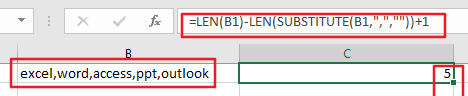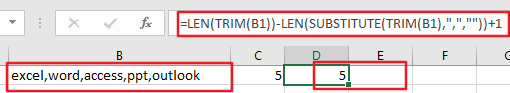### Related Functions

• Excel Substitute function
The Excel SUBSTITUTE function replaces a new text string for an old text string in a text string.The syntax of the SUBSTITUTE function is as below:= SUBSTITUTE  (text, old_text, new_text,[instance_num])….
• Excel LEN function
The Excel LEN function returns the length of a text string (the number of characters in a text string).The syntax of the LEN function is as below:= LEN(text)…
• Excel TRIM function
The Excel TRIM function removes all spaces from text string except for single spaces between words.  You can use the TRIM function to remove extra spaces between words in a string.The syntax of the TRIM function is as below:= TRIM (text)….

## Remove Trailing Spaces from Cells in Excel

This post will guide you how to remove leading and trailing spaces in cells in Excel. How do I remove leading or trailing spaces in a range of cells with a formula in Excel. How to remove trailing spaces from cells with VBA Macro in Excel.

## Remove Trailing Spaces from Cells with Formula

If you want to remote all leading and trailing spaces from test string in one or more cells, you can use a formula based on the TRIM function.

For example, you have a list of data in range B1:B4 contain text string values, and you want to strip all leading and trailing spaces in the given range of cells, how to achieve it. You can use the following formula.

`=TRIM(B1)`

Type this formula into a blank cell and then press Enter key, and drag the AutoFill Handle over other cells to apply this formula.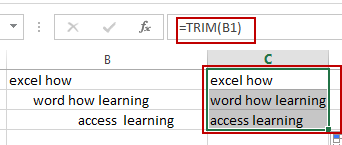This formula will remove both leading and trailing spaces from text string in cells.

## Remove Trailing Spaces from Cells with VBA Macro

You can also use an Excel VBA Macro to remote all trailing spaces from a given range of cells. Just do the following steps:

#1 select one range of cells that you want to remove trailing spaces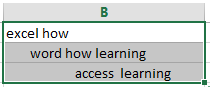#2 open your excel workbook and then click on “Visual Basic” command under DEVELOPER Tab, or just press “ALT+F11” shortcut.#3 then the “Visual Basic Editor” window will appear.

#4 click “Insert” ->”Module” to create a new module.#5 paste the below VBA code into the code window. Then clicking “Save” button.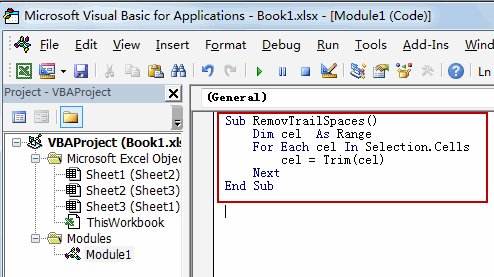```Sub RemovTrailSpaces()
Dim cel  As Range
For Each cel In Selection.Cells
cel = Trim(cel)
Next
End Sub```

#6 back to the current worksheet, then run the above excel macro. Click Run button.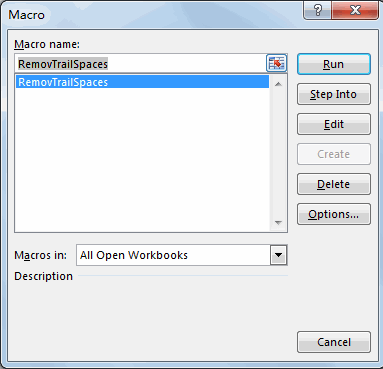#7 Let’s see the result.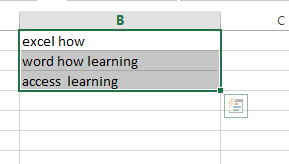### Related Functions

• Excel TRIM function
The Excel TRIM function removes all spaces from text string except for single spaces between words.  You can use the TRIM function to remove extra spaces between words in a string.The syntax of the TRIM function is as below:= TRIM (text)….

## Convert Scientific Notation to Text or a Number in Excel

This post will guide you how to convert scientific notation to text or a number in Excel. How do I convert excel scientific to text with a formula in Excel.

## Convert Scientific Notation to Text or a Number in Excel

Assuming that you have a list of data in range B1:B4 that contain scientific notation numbers and you want to convert those scientific number to text or an integer number. How to achieve it.

You can use the Format cell feature to achieve the result. Just do the following steps:

#1 select the range of cells that you want to convert.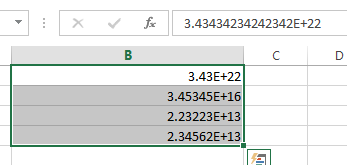#2 right click on it, and select Format Cells from the pop-up menu list. And the Format Cells dialog will open.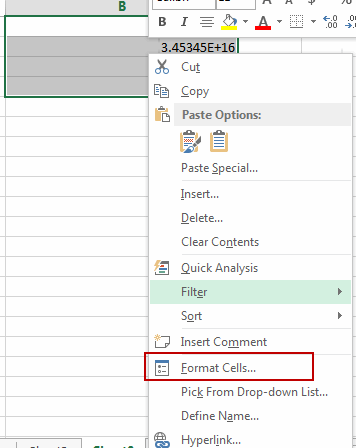#3 switch to Number tab, click Custom under Category list box. And type 0 in the type text box. Then click OK button.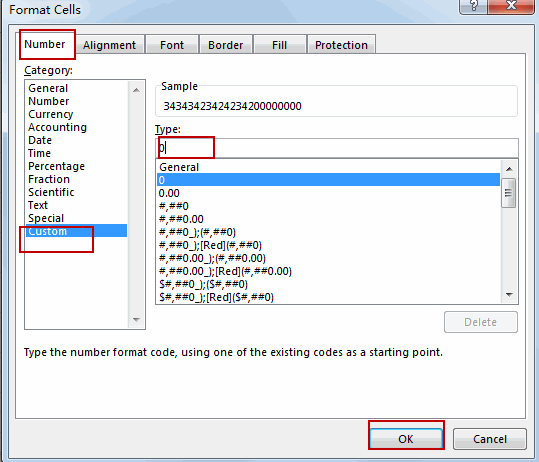#4 Let’s see the result: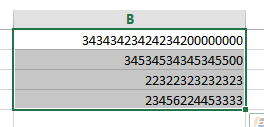You can also use an excel formula to convert scientific notation to text based on the TRIM function or the UPPER function.

Type the following formula into a blank cell and then press Enter key.

=TRIM(B1)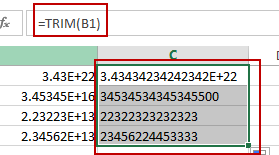Or

=UPPER(B1)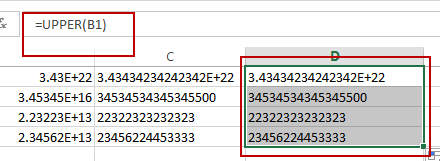There is a simple method to convert scientific notation to text, you just need to type a single quote before the scientific notation number. Then it will show the normal number.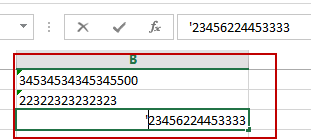### Related Functions

• Excel TRIM function
The Excel TRIM function removes all spaces from text string except for single spaces between words.  You can use the TRIM function to remove extra spaces between words in a string.The syntax of the TRIM function is as below:= TRIM (text)….
• Excel UPPER function
The Excel UPPER function converts all characters in text string to uppercase.The UPPER function is a build-in function in Microsoft Excel and it is categorized as a Text Function.The syntax of the UPPER function is as below:= UPPER (text)…

## Remove Spaces from a String

This post will guide you how to remove extra spaces between words or text string from the specified range of cells in excel. How to delete all spaces from excel cells. For example, when you paste data from an external source to your current worksheet, and you must want to delete all the extra spaces, such as: leading spaces or trailing spaces, or you just want to remote all spaces between words.

## Remove All Spaces from a String

If you want to remove all blanks or spaces in range of cells B1:B4, you can create a formula using SUBSTITUTE function to achieve the result. Like this:

`=SUBSTITUTE(B1," ", "")`

Type this formula in the Cell C1, and press Enter key, then drag the AutoFill Handle over the cells that you want to apply this formula. You will see that all spaces have been removed.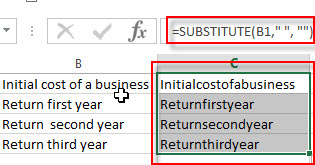Remove All Spaces from a String

If you want to remove extra spaces from text string in cells, you just need to use the TRIM function to remove extra leading space or trailing space or other extra spaces. Type the following formula in cell C1, and press enter key, then drag the AutoFill Handle over the cells that you want to remove extra spaces.

`=TRIM(B1)`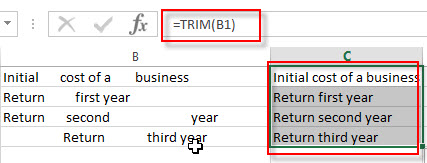### Related Functions

• Excel Substitute function
The Excel SUBSTITUTE function replaces a new text string for an old text string in a text string.The syntax of the SUBSTITUTE function is as below:= SUBSTITUTE  (text, old_text, new_text,[instance_num])….
• Excel TRIM function
The Excel TRIM function removes all spaces from text string except for single spaces between words.  You can use the TRIM function to remove extra spaces between words in a string.The syntax of the TRIM function is as below:= TRIM (text)….

### Related Formulas

• Remove Leading and Trailing Spaces
To remove leading and trailing spaces from text string in Cells, you can use the TRIM function to remove extra spaces.If you want to quickly remove leading and trailing spaces, you can write an  Excel VBA macro code…

## Remove Leading and Trailing Spaces

In this tutorial you will learn that how to remove leading or trailing spaces from a text string in a cell in Excel. This post will guide you how to delete leading or trailing spaces of all cells in a column.

If you remove leading spaces in cells one by one by manually, it will waste a lot of time. So this post will show you two methods to quickly remove leading and trailing spaces from text in one or more cells in your worksheet.

Assuming that you need to remove all leading spaces and trailing spaces in the range of cells A1:A4, you can refer to the following two methods to achieve it.

## Method 1: Remove Leading and Trailing spaces with Excel Formula

To remove leading and trailing spaces from text string in Cells, you can use the TRIM function to remove extra spaces. So you can type the following formula in Cell B1, then press Enter key.

`=TRIM(A1)`

Then select Cell B1 and drag the AutoFill Handle down to other cells that you want to remove the leading or trailing spaces.

You will see that all of your leading and trailing spaces in range A1:A4 are cleaned up.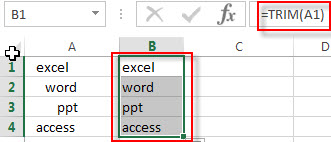Note: if the text contains non-braking spaces, this formula will not work, because the TRIM function is not able to remove non-breaking spaces. At this time, you should remove the non-breaking spaces firstly with the SUBSTITUTE function, and then use the TRIM function to remove the rest leading and trailing spaces. So you can write down the following Excel formula:

`=TRIM(SUBSTITUTE(A1,CHAR(160)," "))`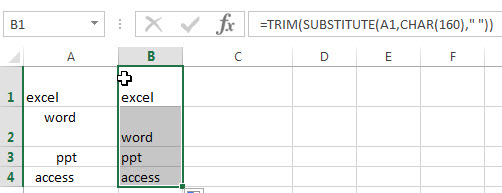You will see that all of leading and trailing spaces are removed from the text string in range A1:A4.

Or try to use the following formula:

`=TRIM(CLEAN(SUBSTITUTE(A1,CHAR(160)," ")))`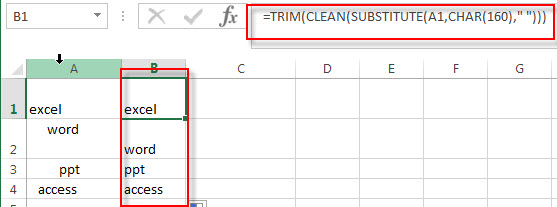The CHAR(160) formula returns a non-breaking space character.

The SUBSTITUTE function will replace all non-breaking space with new space character.

The CLEAN function will remove all non-printing characters, such as line breaks.

## Method 2: Remove Leading and Trailing spaces with Excel VBA macro

If you want to quickly remove leading and trailing spaces, you can write an  Excel VBA macro code, just do it following:

1# click on “Visual Basic” command under DEVELOPER Tab.2# then the “Visual Basic Editor” window will appear.

3# click “Insert” ->”Module” to create a new module4# paste the below VBA code into the code window. Then clicking “Save” button.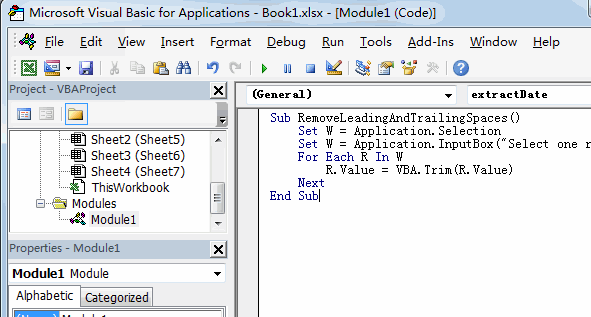```Sub RemoveLeadingAndTrailingSpaces()
Set W = Application.Selection
Set W = Application.InputBox("Select one range that you want to remove leading and trailing spaces:", "RemoveLeadingAndTrailingSpaces", W.Address, Type:=8)
For Each R In W
R.Value = VBA.Trim(R.Value)
Next
End Sub```

5# back to the current worksheet, then run the above excel macro.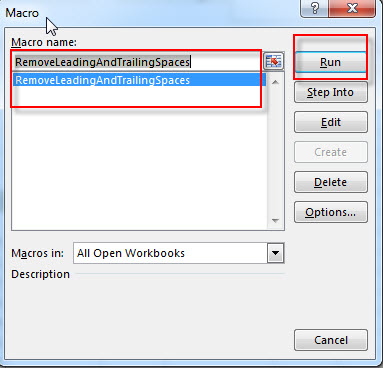6# select the range of cells that you want to remove leading and trailing spaces, then click OK button.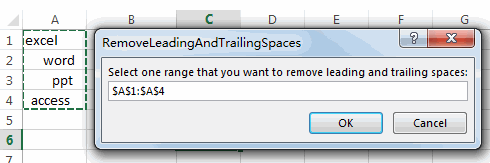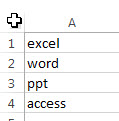### Related Formulas

• count specific words in a cell or a range
If you want to count the number of a specific word in a single cell, you need to use the SUBSTITUTE function to remove all that certain word in text string, then using LEN function to calculate the length of the substring that without that specific word.…
• Extract word that starting with a specific character
Assuming that you have a text string that contains email address in Cell B1, and if you want to extract word that begins with a specific character “@” sign, you can use a combination with the TRIM function, the LEFT function, the SUBSTITUTE function, the MID function, the FIND function, the LEN function and the REPT function to create an excel formula.…
• Extract word that containing a specific character
If you want to extract word that contains a hash character in a text string in Cell B1, you can use a combination of the TRIM function, the MID function, the SUBSTITUTE function, the REPT function, the FIND function to create an excel formula.…
• Count the number of words in a cell
If you want to count the number of words in a single cell, you can create an excel formula based on the IF function, the LEN function, the TRIM function and the SUBSTITUTE function. ..
• Remove Spaces from a String
how to remove extra spaces between words or text string from the specified range of cells in excel. How to delete all spaces from excel cells. …

### Related Functions

• Excel Substitute function
The Excel SUBSTITUTE function replaces a new text string for an old text string in a text string.The syntax of the SUBSTITUTE function is as below:= SUBSTITUTE  (text, old_text, new_text,[instance_num])….
• Excel CHAR function
The Excel CHAR function returns the character specified by a number (ASCII Value).The CHAR function is a build-in function in Microsoft Excel and it is categorized as a Text Function. The syntax of the CHAR function is as below:=CHAR(number)….
• Excel TRIM function
The Excel TRIM function removes all spaces from text string except for single spaces between words.  You can use the TRIM function to remove extra spaces between words in a string.The syntax of the TRIM function is as below:= TRIM (text)….
• Excel CLEAN function
The Excel CLEAN function removes all non-printable characters from a text string.The CLEAN function is a build-in function in Microsoft Excel and it is categorized as a Text Function.The syntax of the CLEAN function is as below:= CLEAN  (text)...

## How to extract nth word from text string

This post will guide you how to extract the nth word from a text string in excel. How do I get the nth word from text string in a cell using excel formula. How to write a User Defined Function to extract nth word from text.

## Extract nth word

If you want to extract the nth word from a text string in a single cell, you can create an excel formula based on the TRIM function, the MID function, the SUBSTITUTE function, the REPT function and the LEN function.

You can use the SUBSTITUTE function replaces each space character with a number of space characters returned by the REPT function. The number of space characters should be equal to the overall length of the original text in cell. So you also need to use the LEN function to combine with the REPT function to create those spaces. Then you can use the MID function to extract the nth word and the starting position can get from the formula (N-1)*LEN(B1)+1 , and the num_chars should be equal to the length of the original text.

Assuming that you want to extract the third word from text string in Cell B1, you can write down the following excel formula:

`=TRIM(MID(SUBSTITUTE(B1," ", REPT(" ",LEN(B1))), (3-1)*LEN(B1) +1, LEN(B1)))`

Let’s see how this formula works:

=REPT(” “,LEN(B1))

This formula will return a new text string that contain a number of the space characters and the number of space character is equal to the length of the original text string in Cell B1. Then it goes into the SUBSTITUTE function as its new_text argument.

=SUBSTITUTE(B1,” “, REPT(” “,LEN(B1)))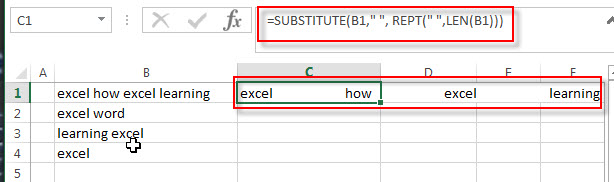This formula replace each space character with new_text that returned by the REPT function. Then it goes into the MID function as its Text argument.

=(3-1)*LEN(B1) +1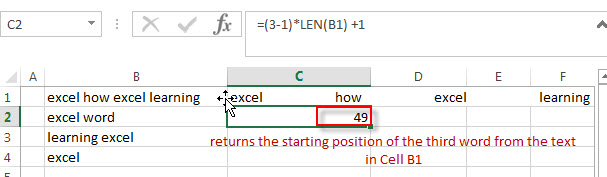This formula returns the starting position of the third word from the text in Cell B1. Then it passed into the MID function as its start_num argument.

=MID(SUBSTITUTE(B1,” “, REPT(” “,LEN(B1))), (3-1)*LEN(B1) +1, LEN(B1))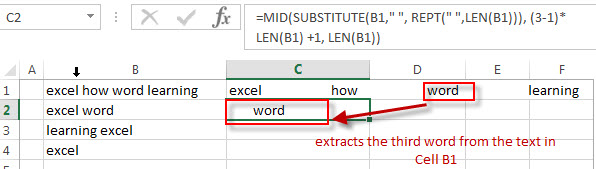The both Text and start_num arguments are returned by the above two formula, so this formula extracts the third word from the text in Cell B1.

=TRIM()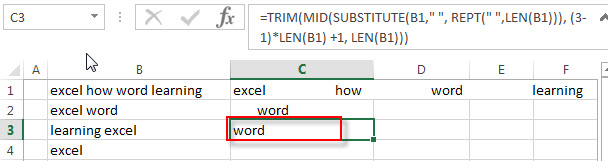The TRIM function removes all extra space characters and just leave only one between words.

## Extract Nth word with User Defined Function

You can also write a User Defined Function to extract the nth word from the text string in a cell in Excel VBA:

1# click on “Visual Basic” command under DEVELOPER Tab.2# then the “Visual Basic Editor” window will appear.

3# click “Insert” ->”Module” to create a new module4# paste the below VBA code into the code window. Then clicking “Save” button.

```Function GetNthWord(Source As String, Position As Integer)
Dim arr() As String
arr = VBA.Split(Source, " ")
xCount = UBound(arr)
If xCount < 1 Or (Position - 1) > xCount Or Position < 0 Then
GetNthWord = ""
Else
GetNthWord = arr(Position - 1)
End If
End Function```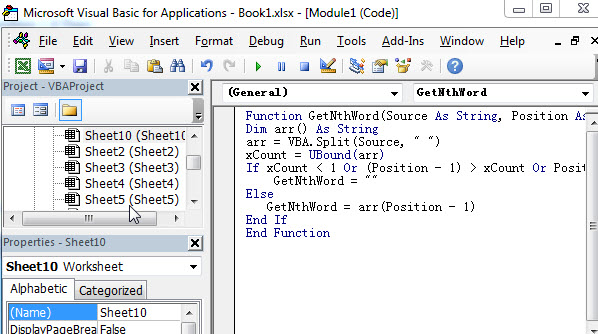5# back to the current worksheet, then enter the below formula in Cell C1: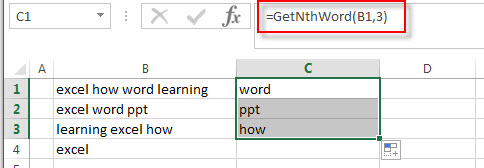### Related Formulas

• count specific words in a cell or a range
If you want to count the number of a specific word in a single cell, you need to use the SUBSTITUTE function to remove all that certain word in text string, then using LEN function to calculate the length of the substring that without that specific word.…
• Get first word from text string
If you want to extract the first word from a text string in a cell, you can use a combination of the IF function, the ISERR function, the LEFT function and the FIND function to create a complex excel formula..…
• Get last word from text string
If you want to get the last word from a text string, you can create an excel formula based on the RIGHT function, the LEN function, the FIND function and the SUBSTITUTE function..…
• Extract word that starting with a specific character
Assuming that you have a text string that contains email address in Cell B1, and if you want to extract word that begins with a specific character “@” sign, you can use a combination with the TRIM function, the LEFT function, the SUBSTITUTE function, the MID function, the FIND function, the LEN function and the REPT function to create an excel formula.…

### Related Functions

• Excel Substitute function
The Excel SUBSTITUTE function replaces a new text string for an old text string in a text string.The syntax of the SUBSTITUTE function is as below:= SUBSTITUTE  (text, old_text, new_text,[instance_num])….
• Excel REPT function
The Excel REPT function repeats a text string a specified number of times.The syntax of the REPT function is as below:= REPT  (text, number_times)…
• Excel TRIM function
The Excel TRIM function removes all spaces from text string except for single spaces between words.  You can use the TRIM function to remove extra spaces between words in a string.The syntax of the TRIM function is as below:= TRIM (text)….
• Excel MID function
The Excel MID function returns a substring from a text string at the position that you specify.The syntax of the MID function is as below:= MID (text, start_num, num_chars)….
• Excel LEN function
The Excel LEN function returns the length of a text string (the number of characters in a text string).The syntax of the LEN function is as below:= LEN(text)…

## How to count the number of words in a range of cells in Excel

In the previous post, we talked that how to count the number of words in a single cell. And this post explains that how to count the number of words in a range of cells using excel formula. And how to count the total words in a range of cell with VBA Code.

## Count the number of words in a range of cells

If you want to count the number of words in a range of cell, you need to use a combination of the SUMPRODUCT function, the IF function, the LEN function, the TRIM function and the SUBSTITUTE function to create an excel formula.

You need to use the SUBSTITUTE function to remove all spaces from the text for each cell in the range, then using LEN function to calculate the length of the text without spaces. The returned number is subtracted from the length of the text string with space characters to get the number of spaces in the text. And then add 1 to get the number of words in the range.

Assuming that you want to count the number of words in the range B1:B3, you can write down an excel array formula as follows:

`=SUMPRODUCT(IF(LEN(TRIM(B1:B3))=0, 0, LEN(TRIM(B1:B3))-LEN(SUBSTITUTE(B1:B3," ", ""))+1))`

Let’s see how this formula works:

=LEN(TRIM(B1:B3))-LEN(SUBSTITUTE(B1:B3,” “, “”))+1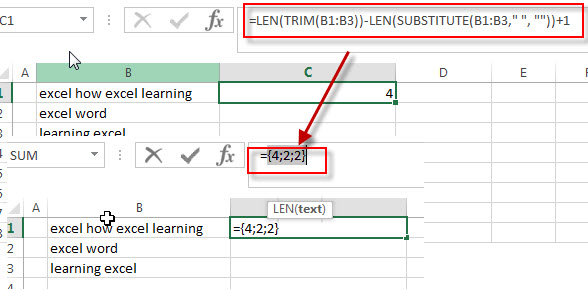This formula returns an array result that contains a number of words for each cell in the range. The array result is like this: {4;2;2}, then it goes into the IF function as its argument.

=IF(LEN(TRIM(B1:B3))=0, 0, LEN(TRIM(B1:B3))-LEN(SUBSTITUTE(B1:B3,” “, “”))+1)

The IF function will check if it is an empty cell, if TRUE, then returns 0, otherwise, returns the number of words in the text in cell.

=SUMPRODUCT()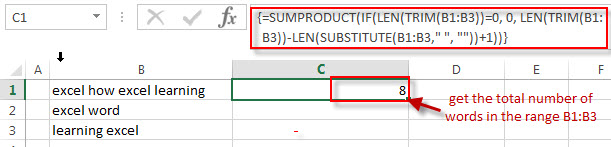This formula will sum all items in that array result that returned by the IF formula to get the total number of words in the range B1:B3.

## Count the number of words in a range of cells with VBA Code

You can also create a new user defined function to count the number of words in a range in Excel VBA:

1# click on “Visual Basic” command under DEVELOPER Tab.2# then the “Visual Basic Editor” window will appear.

3# click “Insert” ->”Module” to create a new module4# paste the below VBA code into the code window. Then clicking “Save” button.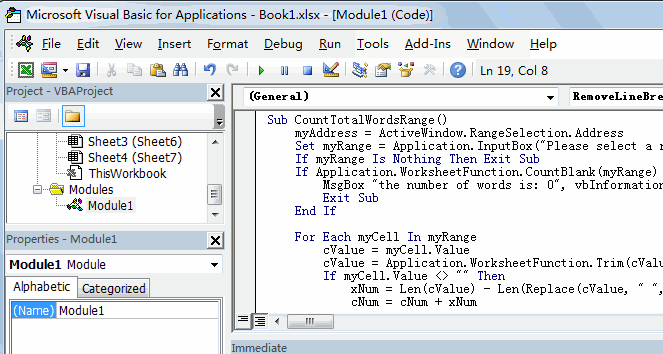```Sub CountTotalWordsRange()
Set myRange = Application.InputBox("Please select a range that you want to count words:", "CountTotalWordsRange", myAddress, , , , , 8)
If myRange Is Nothing Then Exit Sub
If Application.WorksheetFunction.CountBlank(myRange) = myRange.Count Then
MsgBox "the number of words is: 0", vbInformation, "CountTotalWordsRange"
Exit Sub
End If

For Each myCell In myRange
cValue = myCell.Value
cValue = Application.WorksheetFunction.Trim(cValue)
If myCell.Value <> "" Then
xNum = Len(cValue) - Len(Replace(cValue, " ", "")) + 1
cNum = cNum + xNum
End If
Next myCell
MsgBox "the number of words Is: " & Format(cNum, "#,##0"), vbOKOnly, "CountTotalWordsRange"
End Sub```

5# back to the current worksheet, then run the above excel macro, then select a range that you want to count the number of words.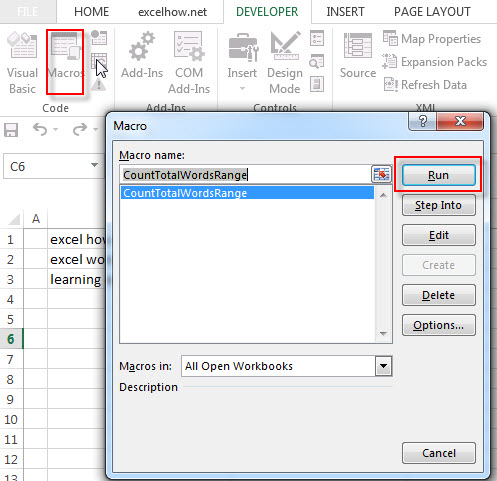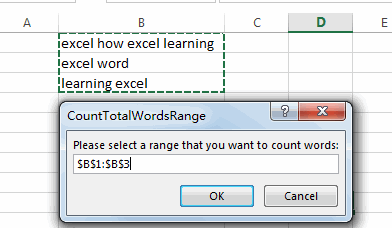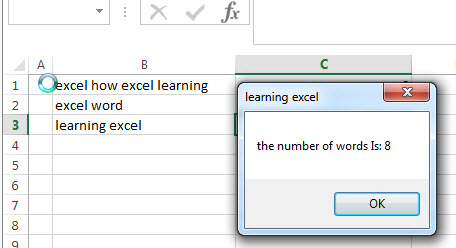### Related Formulas

• count specific words in a cell or a range
If you want to count the number of a specific word in a single cell, you need to use the SUBSTITUTE function to remove all that certain word in text string, then using LEN function to calculate the length of the substring that without that specific word.…
• Count the number of words in a cell
If you want to count the number of words in a single cell, you can create an excel formula based on the IF function, the LEN function, the TRIM function and the SUBSTITUTE function. ..
• Extract word that starting with a specific character
Assuming that you have a text string that contains email address in Cell B1, and if you want to extract word that begins with a specific character “@” sign, you can use a combination with the TRIM function, the LEFT function, the SUBSTITUTE function, the MID function, the FIND function, the LEN function and the REPT function to create an excel formula.…

### Related Functions

• Excel SUMPRODUCT function
The Excel SUMPRODUCT function multiplies corresponding components in the given one or more arrays or ranges, and returns the sum of those products.The syntax of the SUMPRODUCT function is as below:= SUMPRODUCT (array1,[array2],…)…
• Excel Substitute function
The Excel SUBSTITUTE function replaces a new text string for an old text string in a text string.The syntax of the SUBSTITUTE function is as below:= SUBSTITUTE  (text, old_text, new_text,[instance_num])….
• Excel IF function
The Excel IF function perform a logical test to return one value if the condition is TRUE and return another value if the condition is FALSE. The IF function is a build-in function in Microsoft Excel and it is categorized as a Logical Function.The syntax of the IF function is as below:= IF (condition, [true_value], [false_value])….
• Excel LEN function
The Excel LEN function returns the length of a text string (the number of characters in a text string).The syntax of the LEN function is as below:= LEN(text)…
• Excel TRIM function
The Excel TRIM function removes all spaces from text string except for single spaces between words. The syntax of the TRIM function is as below:=TRIM(text)…

## How to count the number of words in a cell in Excel

This post explains that how to count the number of words in a cell using excel formula. How to count total words in a cell with User Defined Functions in excel VBA.

## Count the number of words in a cell

If you want to count the number of words in a single cell, you can create an excel formula based on the IF function, the LEN function, the TRIM function and the SUBSTITUTE function. You need to use the SUBSTITUTE function to remove all space strings from the text string in a cell, then the returned value goes into the LEN function to get the length of the text without space string. You still need to get the length of the text with space strings and then subtract to the length of the text without space to get the number of spaces, then add 1 to get the number of words in a cell.

Assuming that you want to get the number of words in cell B1, you can write down an excel formula as follows:

`=IF(LEN(TRIM(B1))=0, 0, LEN(TRIM(B1))-LEN(SUBSTITUTE(B1," ", ""))+1)`

Let’s see how this formula works:

=LEN(TRIM(B1))-LEN(SUBSTITUTE(B1,” “, “”))+1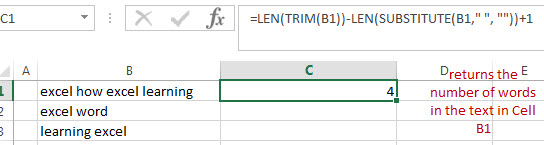This formula returns the number of words in the text in Cell B1. The SUBSTITUTE function replaces all space strings with empty string to remove all spaces, and using LEN function to get the length of the text without spaces.

The TRIM function will remove extra spaces from the text and just leave one space between words.

=IF(LEN(TRIM(B1))=0, 0, LEN(TRIM(B1))-LEN(SUBSTITUTE(B1,” “, “”))+1)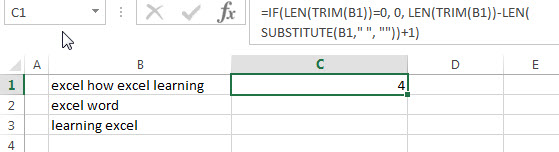The IF function will check if it is an empty cell, if TRUE, then returns 0, otherwise, returns the number of words in the text in cell.

## Count the number of words in a cell with User Defined Function

You can also create a new user defined function to count the number of words in a cell in Excel VBA:

1# click on “Visual Basic” command under DEVELOPER Tab.2# then the “Visual Basic Editor” window will appear.

3# click “Insert” ->”Module” to create a new module4# paste the below VBA code into the code window. Then clicking “Save” button.

```Function countTotalWordCell(rng As Range) As Integer
countTotalWordCell = UBound(Split(rng.Value, " "), 1) + 1
End Function```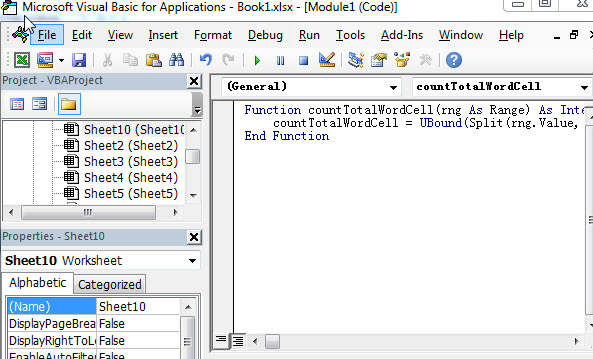5# back to the current worksheet, then enter the below formula in Cell C1: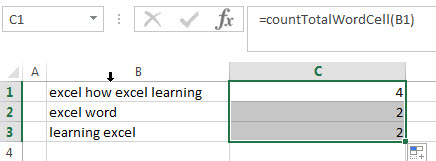### Related Formulas

• count specific words in a cell or a range
If you want to count the number of a specific word in a single cell, you need to use the SUBSTITUTE function to remove all that certain word in text string, then using LEN function to calculate the length of the substring that without that specific word.…
• Extract word that starting with a specific character
Assuming that you have a text string that contains email address in Cell B1, and if you want to extract word that begins with a specific character “@” sign, you can use a combination with the TRIM function, the LEFT function, the SUBSTITUTE function, the MID function, the FIND function, the LEN function and the REPT function to create an excel formula.…

### Related Functions

• Excel Substitute function
The Excel SUBSTITUTE function replaces a new text string for an old text string in a text string.The syntax of the SUBSTITUTE function is as below:= SUBSTITUTE  (text, old_text, new_text,[instance_num])….
• Excel IF function
The Excel IF function perform a logical test to return one value if the condition is TRUE and return another value if the condition is FALSE. The IF function is a build-in function in Microsoft Excel and it is categorized as a Logical Function.The syntax of the IF function is as below:= IF (condition, [true_value], [false_value])….
• Excel LEN function
The Excel LEN function returns the length of a text string (the number of characters in a text string).The syntax of the LEN function is as below:= LEN(text)…
• Excel TRIM function
The Excel TRIM function removes all spaces from text string except for single spaces between words. The syntax of the TRIM function is as below:=TRIM(text)…

## How to extract word that containing a specific character

This post will guide you how to extract word that containing a specific character using formula in excel. How to get a word that contains a specific character (such as: @, comma, hash, etc.) in a text string in one cell.

## Extract word that containing a specific character

If you want to extract word that contains a hash character in a text string in Cell B1, you can use a combination of the TRIM function, the MID function, the SUBSTITUTE function, the REPT function, the FIND function to create an excel formula as follows:

`=TRIM(MID(SUBSTITUTE(B1," ",REPT(" ",255)),FIND("#",SUBSTITUTE(B1," ",REPT(" ",255)))-100,255))`

Let’s see how this formula works:

= SUBSTITUTE(B1,” “,REPT(” “,255))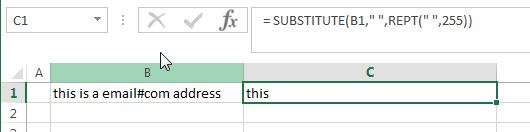The REPT function will return a new text string that contains 255 empty spaces.  And it goes into the SUBSTITUTE function as its new_text argument.  Then the SUBSTITUTE function will replace all empty string with new text value returned by the REPT function.

= FIND(“#”,SUBSTITUTE(B1,” “,REPT(” “,255)))-100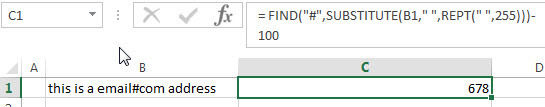This formula will locate the position of the first hash character (#) in a text string that returned by the SUBSTITUTE function.

= MID(SUBSTITUTE(B1,” “,REPT(” “,255)),FIND(“#”,SUBSTITUTE(B1,” “,REPT(” “,255)))-100,255)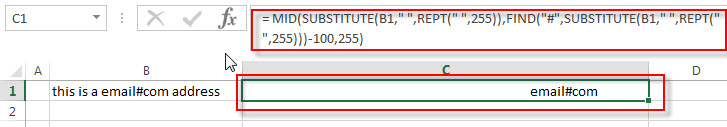This MID function will extract 255 characters from a text string (returned by the SUBSTITUTE function) at a specific position that returned by the FIND function. Then the word that contain hash character will be returned.

=TRIM()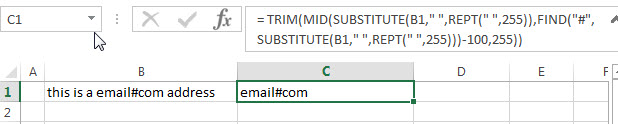The TRIM function removes all spaces from text string returned by the MID function, just leave one space between words.

### Related Formulas

• Split Multiple Lines from a Cell into Rows
If you have multiple lines in a cell and each line is separated by line break character or press “alt + enter” key while you entering the text string into cells, and you need to extract the multiple lines into the separated rows or columns, you can use a combination with the TRIM function, the MID function, the SUBSTITUTE function, the REPT function, the LEN function to create a complex excel formula..…
• Extract word that starting with a specific character
Assuming that you have a text string that contains email address in Cell B1, and if you want to extract word that begins with a specific character “@” sign, you can use a combination with the TRIM function, the LEFT function, the SUBSTITUTE function, the MID function, the FIND function, the LEN function and the REPT function to create an excel formula.…

### Related Functions

• Excel Substitute function
The Excel SUBSTITUTE function replaces a new text string for an old text string in a text string.The syntax of the SUBSTITUTE function is as below:= SUBSTITUTE  (text, old_text, new_text,[instance_num])….
• Excel TRIM function
The Excel TRIM function removes all spaces from text string except for single spaces between words.  You can use the TRIM function to remove extra spaces between words in a string.The syntax of the TRIM function is as below:= TRIM (text)….
• Excel MID function
The Excel MID function returns a substring from a text string at the position that you specify.The syntax of the MID function is as below:= MID (text, start_num, num_chars)….
• Excel FIND function
The Excel FIND function returns the position of the first text string (sub string) within another text string.The syntax of the FIND function is as below:= FIND(find_text, within_text,[start_num])…
• Excel REPT function
The Excel REPT function repeats a text string a specified number of times.The syntax of the REPT function is as below:= REPT  (text, number_times)…

## How to extract word that starting with a specific character

This post will guide you how to extract a word that starts with a specific character in excel.

## Extract word that starting with a specific character

For example, Assuming that you have a text string that contains email address in Cell B1, and if you want to extract word that begins with a specific character “@” sign, you can use a combination with the TRIM function, the LEFT function, the SUBSTITUTE function, the MID function, the FIND function, the LEN function and the REPT function to create an excel formula as follows:

`=TRIM(LEFT(SUBSTITUTE(MID(B1, FIND("@",B1), LEN(B1))," ",REPT(" ",LEN(B1))),LEN(B1)))`

Let’s see how this formula works:

=LEN(B1)

The LEN function returns the number of characters in a text string in Cell B1. The returned result goes into the MID function as its num_chars argument.

= FIND(“@”,B1)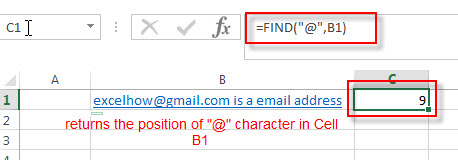The FIND function returns the position of the first specific character “@” sign in Cell B1. It returns 9. It goes into the MID function as its start_num argument.

=MID(B1, FIND(“@”,B1), LEN(B1))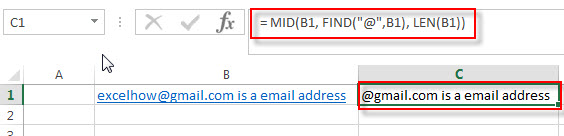The MID function extracts a substring at the starting position (returned by the FIND function) and num_chars value (returned by LEN function)

=REPT(” “,LEN(B1))

This formula repeats empty string a specified number of times returned by the LEN function. It will go into the SUBSTITUTE function as its new_text argument.

= SUBSTITUTE(MID(B1, FIND(“@”,B1), LEN(B1)),” “,REPT(” “,LEN(B1)))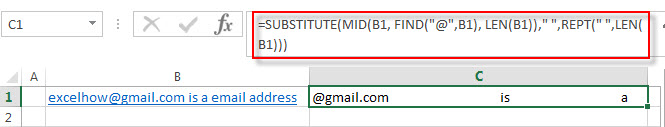The SUBSTITUTE function will replace all empty string with another new text string returned by the REPT function from a text string returned by the MID function.  Then the returned value goes into the LEFT function as its Text argument.

= LEFT(SUBSTITUTE(MID(B1, FIND(“@”,B1), LEN(B1)),” “,REPT(” “,LEN(B1))),LEN(B1))The LEFT function extracts a specified number of the characters from a text string returned by the SUBSTITUTE function, starting from the leftmost character and the num_chars value is equal to the length of the string in Cell B1.

=TRIM()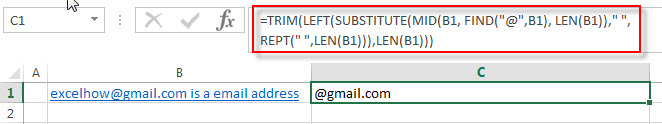The TRIM function removes all spaces from text string returned by the LEFT function, just leave one space between words.

### Related Formulas

• Split Multiple Lines from a Cell into Rows
If you have multiple lines in a cell and each line is separated by line break character or press “alt + enter” key while you entering the text string into cells, and you need to extract the multiple lines into the separated rows or columns, you can use a combination with the TRIM function, the MID function, the SUBSTITUTE function, the REPT function, the LEN function to create a complex excel formula..…
• Extract word that containing a specific character
If you want to extract word that contains a hash character in a text string in Cell B1, you can use a combination of the TRIM function, the MID function, the SUBSTITUTE function, the REPT function, the FIND function to create an excel formula.…

### Related Functions

• Excel Substitute function
The Excel SUBSTITUTE function replaces a new text string for an old text string in a text string.The syntax of the SUBSTITUTE function is as below:= SUBSTITUTE  (text, old_text, new_text,[instance_num])….
• Excel TRIM function
The Excel TRIM function removes all spaces from text string except for single spaces between words.  You can use the TRIM function to remove extra spaces between words in a string.The syntax of the TRIM function is as below:= TRIM (text)….
• Excel LEFT function
The Excel LEFT function returns a substring (a specified number of the characters) from a text string, starting from the leftmost character.The syntax of the LEFT function is as below:= LEFT(text,[num_chars])….
• Excel MID function
The Excel MID function returns a substring from a text string at the position that you specify.The syntax of the MID function is as below:= MID (text, start_num, num_chars)….
• Excel FIND function
The Excel FIND function returns the position of the first text string (sub string) within another text string.The syntax of the FIND function is as below:= FIND(find_text, within_text,[start_num])…
• Excel LEN function
The Excel LEN function returns the length of a text string (the number of characters in a text string).The syntax of the LEN function is as below:= LEN(text)…
• Excel REPT function
The Excel REPT function repeats a text string a specified number of times.The syntax of the REPT function is as below:= REPT  (text, number_times)…

## How to join text from two or more cells into one cell separated by commas, space

This post explains that how to join multiple cells into one cell with commas or space character in excel.  How to combine text from two or more cells into one cell with space, commas or others specific characters as delimiter.

## Join text from two or more cells with commas

You can merge text from two or more cells into one cell using a combination of the SUBSTITUTE function, the TRIM function and concatenation operator to create an excel formula.

For example, if you just want to combine cells from a range A1:C1 to a cell with commas, just use concatenation operator as follows:

`=A1&", "&B1&", "&C1`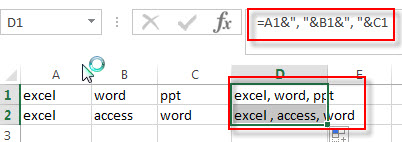If some of values in Cells are empty, you can use the concatenation operator to join text from all cells, and then you need to use TRIM function to remove all space character from text string except for single space between

All words. Then you can use the SUBSTITUTE function to replace all empty strings with comma.

So you can use the following excel formula:

`=SUBSTITUTE(TRIM(A1&" "&B1&" "&C1))`

Let’s see how this formula works:

= A1&” “&B1&” “&C1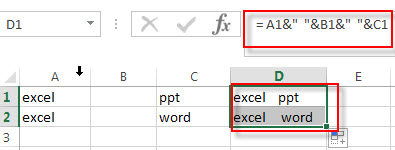You will see that there are multiple empty string between words.

=TRIM(A1&” “&B1&” “&C1)This formula removes empty strings from the text string and just leaves one space between all words.

=SUBSTITUTE(TRIM(A1&” “&B1&” “&C1),” “,”,”)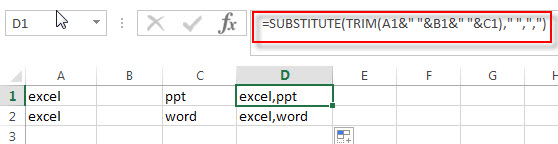The SUBSTITUTE function replaces all space characters with commas.

### Related Formulas

• Split Multiple Lines from a Cell into Rows
If you have multiple lines in a cell and each line is separated by line break character or press “alt + enter” key while you entering the text string into cells, and you need to extract the multiple lines into the separated rows or columns, you can use a combination with the TRIM function, the MID function, the SUBSTITUTE function, the REPT function, the LEN function to create a complex excel formula..…
• The difference between Replace function and Substitute function in Excel
There are two similar functions to replace text string in Excel. They are REPLACE and SUBSTITUTE functions. What’s the difference between REPLACE function and SUBSTITUTE function in excel 2013? .…

### Related Functions

• Excel Substitute function
The Excel SUBSTITUTE function replaces a new text string for an old text string in a text string.The syntax of the SUBSTITUTE function is as below:= SUBSTITUTE  (text, old_text, new_text,[instance_num])….
• Excel TRIM function
The Excel TRIM function removes all spaces from text string except for single spaces between words.  You can use the TRIM function to remove extra spaces between words in a string.The syntax of the TRIM function is as below:= TRIM (text)….